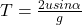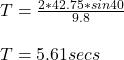A baseball is hit by a batter with an initial velocity of 95 miles per hour at 45° above the horizontal. The opposing team misses the catch

Question

A baseball is hit by a batter with an initial velocity of 95 miles per hour at 45° above the horizontal. The opposing team misses the catch and the baseball lands in the outfield 50 meters from where it started. How long was the baseball in the air

in progress 0
5 months 2021-08-27T13:01:19+00:00 1 Answers 2 views 0

5.61 seconds

Explanation:

The baseball moves as a projectile. Its angle is 45° and its velocity is 95 miles per hour.

Let us convert this to metres per second:

1 mile per hour = 0.45 m/s

95 miles per hour = 95 * 0.45 = 42.75 m/s

We need to find the time of flight of the baseball. Time of flight is given as:where u = initial velocity

α = angle

g = acceleration due to gravity (9.8 m/s)

Therefore:The baseball was in the air for 5.61 seconds.